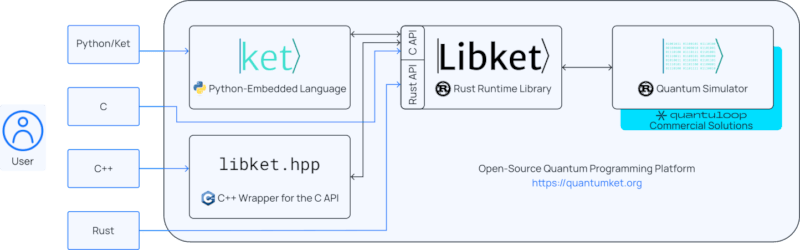## libket

Runtime library for the Ket programming language

### 8 releases

 0.3.1 May 16, 2023 May 15, 2023 Apr 13, 2023 Nov 16, 2022 Jul 14, 2022

#146 in Programming languages

Used in kbw

Apache-2.0

135KB
2.5K SLoC

# Libket

Libket serves as the core of the Ket Quantum Programming platform, providing a runtime library for the quantum programming language Ket, as well as simple interfaces for developing quantum applications in C/C++ and Rust. For more information, please refer to the documentation at https://quantumket.org.

## Ket Quantum Programming platform## Examples

For examples of Libket being used in Rust, C, and C++, check out the Ket Everywhere repository.

# Libket Quantum Programming Library

The Libket library provides a set of tools for quantum programming in Rust. It serves as the runtime library for the Python-embedded quantum programming language Ket.

## Usage

To use this library, add the following line to your `Cargo.toml` file:

``````[dependencies]
libket = "0.3.1"
``````

Additionally, you may need to include the following dependencies for quantum code serialization/deserialization and the KBW quantum computer simulator:

``````serde = { version = "1.0", features = ["derive"] }
serde_json = "1.0"
kbw = "0.1.6"
``````

## Examples

The following example demonstrates the implementation of Grover's algorithm using Libket:

``````use ket::*;

fn main() -> Result<()> {
let n = 4;  // Number of qubits

let p = Process::new_ptr();  // Create a new quantum process

let mut qubits = Quant::new(&p, n)?;  // Create a quantum register with `n` qubits

h(&qubits)?;  // Apply Hadamard gate to all qubits

let steps = (std::f64::consts::PI / 4.0 * f64::sqrt((1 << n) as f64)) as i32;  // Calculate the number of steps for the Grover's algorithm

for _ in 0..steps {
ctrl(&qubits.slice(1..), || z(&qubits.at(0)))??;  // Apply controlled-Z gate with the first qubit as the target and the rest as controls

around(
&p,
|| {
h(&qubits).unwrap();  // Apply Hadamard gate to all qubits
x(&qubits).unwrap();  // Apply Pauli-X gate to all qubits
},
|| ctrl(&qubits.slice(1..), || z(&qubits.at(0))),  // Apply controlled-Z gate with the first qubit as the target and the rest as controls
)???;
}

let _ = measure(&mut qubits)?;  // Perform measurement on the qubits

let mut p = p.borrow_mut();
p.prepare_for_execution()?;  // Prepare the quantum program for execution
println!("{:#?}", p.blocks());  // Print the blocks of the quantum program

Ok(())
}
``````

The example demonstrates the usage of various Libket functions to implement Grover's algorithm, including qubit initialization, gate operations, control structures, and measurement.

For more examples, refer to the Libket Git repository https://gitlab.com/quantum-ket/libket.

~2.2–3MB
~66K SLoC